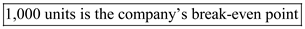# Quiz 5: Cost-Volume-Profit Relationships

Business

1)Contribution Format Income Statement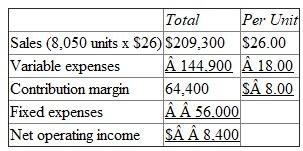2)Contribution Format Income Statement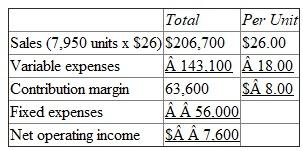3)Contribution Format Income Statement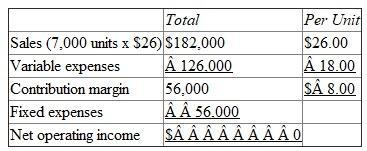4205-6-1Q AID: 0544 | 21/08/2012 RID: 2035 | 30/08/2012 Product's contribution margin ratio is computed by diving contribution margin by sales in dollars. This ratio is presented as percentage of sales. Contribution margin is calculated by deducting variable costs from sales revenue. This is used to meet the fixed costs and provide for profit. Thus it is useful in estimating the sales volume needed for estimated earnings target. It is useful to predict the net income at given sales revenue. Hence it is very useful in business planning.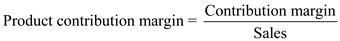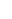1)There are three steps to follow in order to plot a CVP graph: First , to determine the fixed cost, draw a parallel line to the x-axis (volume) at its fixed dollar amount. In this case, \$12,000 Second , determine the total cost. Total cost formula follows the cost formula: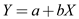Where "a"= fixed costs, "b"= variable costs, and "X"= number of units. Pick the relevant volume, in this case 2,000, and solve for the total cost.Third , determine total sales revenue. Choose the maximum sales level, in this case 2000 units.Now plot each line. Keep in mind the total sales revenue line starts at 0 on the graph while the total costs line starts at the same point the fixed cost line does. See graph below: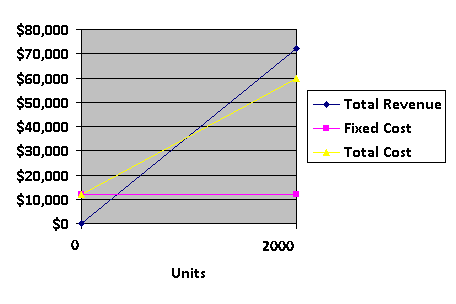2)The break-even point is the point where the total sales revenue and the total expense lines intersect. As illustrated by the red line above, this is about the halfway point between 0 and 2,000 units, which we can estimate as 1,000 units. We can verify this using the CVP formula. The break-even point will yield \$0 of profit: Note: Unit contribution margin = selling price/unit - variable cost / unit Q = Quantity sold